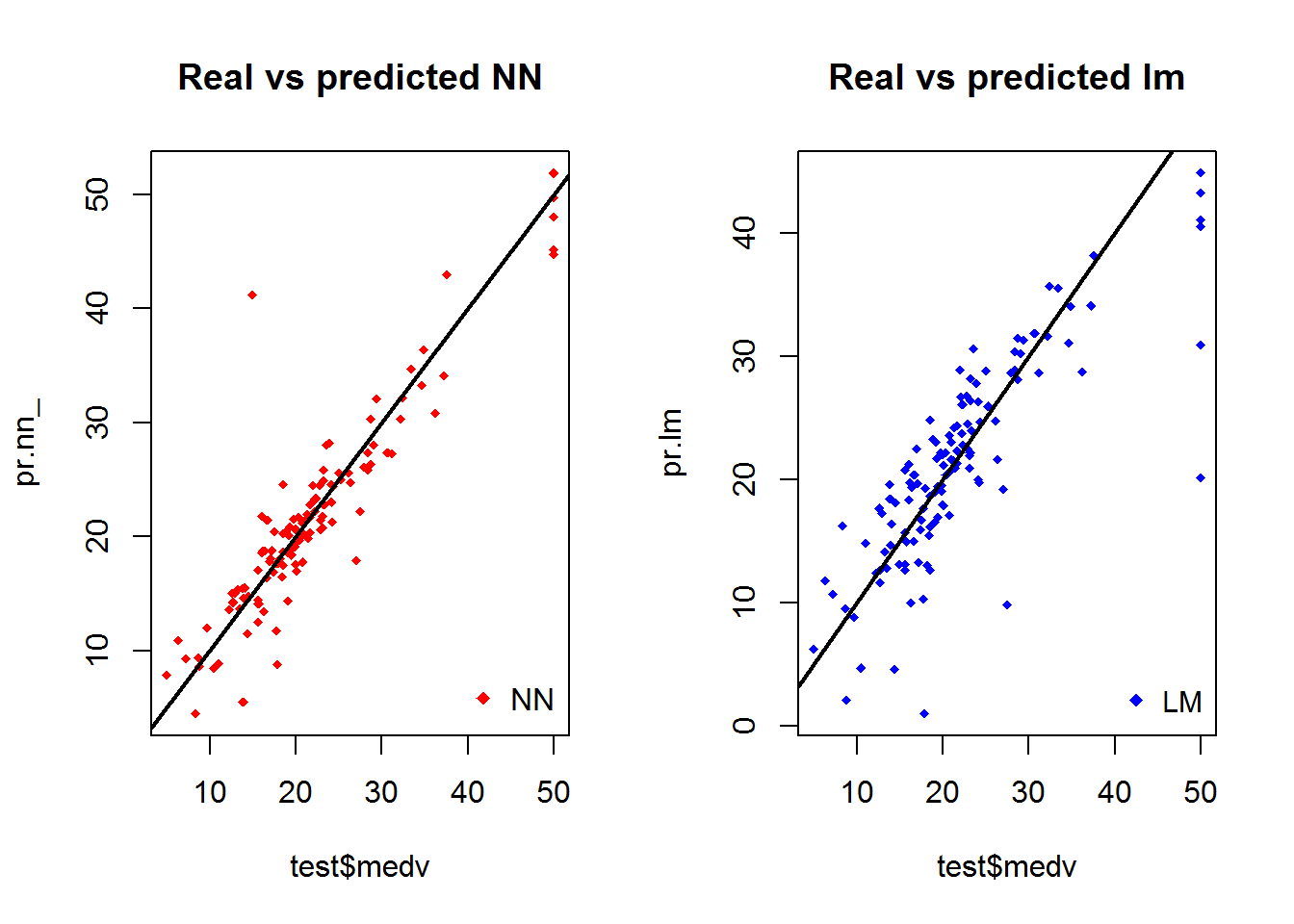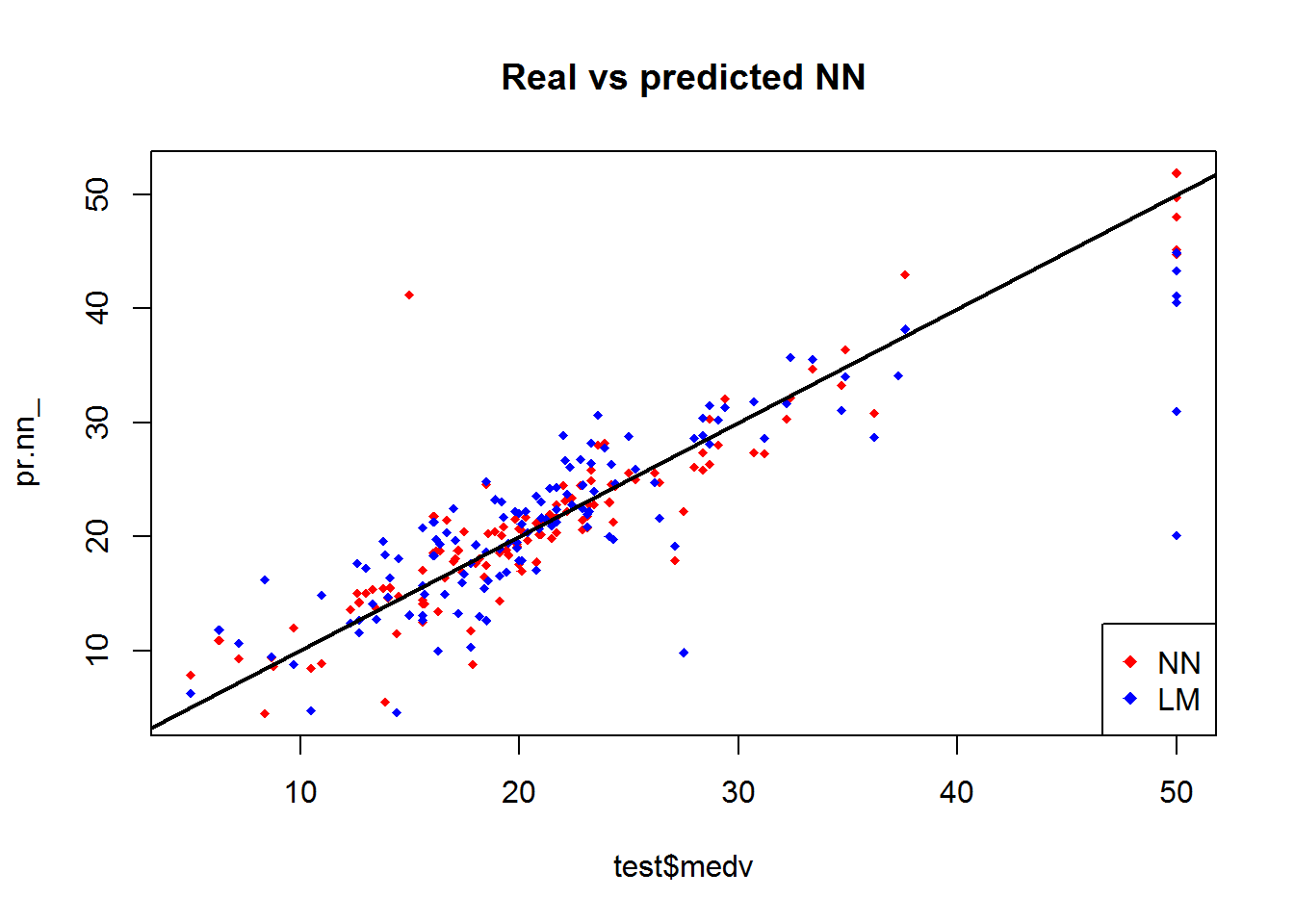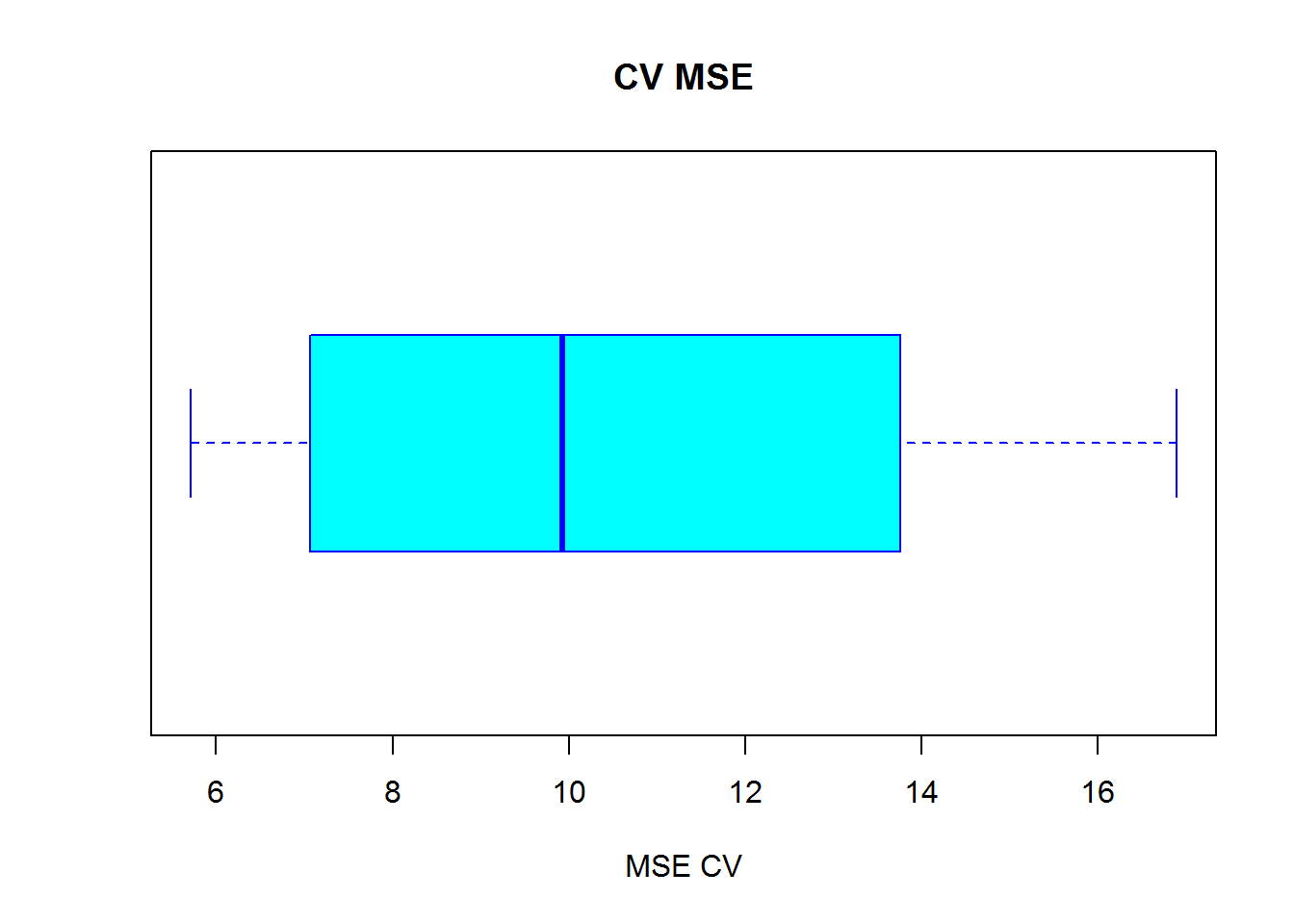## Goal

Fit a simple neural network using neuralnet.

## The Data

Use the Boston dataset in the MASS package. The Boston dataset is a collection of data about housing values in the suburbs of Boston. Predict the median value of homes (medv) using all the other continuous variables available.

data <- Boston

Check for missing values.

apply(data, 2, function(x) sum(is.na(x)))
##    crim      zn   indus    chas     nox      rm     age     dis     rad
##       0       0       0       0       0       0       0       0       0
##     tax ptratio   black   lstat    medv
##       0       0       0       0       0

There is no missing data. Continue splitting the data into train/test sets. Fit a linear regression model. Use mean squared error (MSE) as a measure of prediction preformance.

index <- sample(1:nrow(data), round(0.75 * nrow(data)))
train <- data[index, ]
test <- data[-index, ]
lm.fit <- glm(medv~., data=train)
summary(lm.fit)
##
## Call:
## glm(formula = medv ~ ., data = train)
##
## Deviance Residuals:
##      Min        1Q    Median        3Q       Max
## -17.1991   -2.6672   -0.7363    2.0047   26.5883
##
## Coefficients:
##               Estimate Std. Error t value Pr(>|t|)
## (Intercept)  27.716142   5.856932   4.732 3.18e-06 ***
## crim         -0.114376   0.035129  -3.256 0.001236 **
## zn            0.028660   0.015878   1.805 0.071893 .
## indus         0.010242   0.069561   0.147 0.883026
## chas          2.476606   0.942578   2.627 0.008964 **
## nox         -15.289084   4.414232  -3.464 0.000596 ***
## rm            5.018929   0.487842  10.288  < 2e-16 ***
## age          -0.024900   0.014621  -1.703 0.089413 .
## dis          -1.488864   0.229648  -6.483 2.91e-10 ***
## rad           0.244957   0.073908   3.314 0.001010 **
## tax          -0.011259   0.004157  -2.709 0.007074 **
## ptratio      -0.903044   0.145202  -6.219 1.36e-09 ***
## black         0.008499   0.003167   2.684 0.007606 **
## lstat        -0.420232   0.060202  -6.980 1.39e-11 ***
## ---
## Signif. codes:  0 '***' 0.001 '**' 0.01 '*' 0.05 '.' 0.1 ' ' 1
##
## (Dispersion parameter for gaussian family taken to be 21.5379)
##
##     Null deviance: 32455.3  on 379  degrees of freedom
## Residual deviance:  7882.9  on 366  degrees of freedom
## AIC: 2260.7
##
## Number of Fisher Scoring iterations: 2
pr.lm <- predict(lm.fit, test)
MSE.lm <- sum((pr.lm - test$medv)^2)/nrow(test) MSE.lm ##  27.66452 ### Fit Neural Model #### Data Preprocessing. Normalize your data before training a neural network: avoiding normalization may lead to useless results or to a very difficult training process. There are different methods to scale the data like z-normalization, min-max scale, and others. Use min-max method and scale the data in the interval [0,1]. maxs <- apply(data, 2, max) mins <- apply(data, 2, min) scaled <- as.data.frame(scale(data, center = mins, scale = maxs - mins)) train_ <- scaled[index,] test_ <- scaled[-index,] Note that scale returns a matrix that needs to be coerced into a data frame. #### Parameters Determining the number of layers and neurons do no tlend htemsdelves to widely accepted stadnards. One hidden layer is enough for most of applications. The number of neurons could follow this rough guidance: • input layer size < # neurons < output layer size • typically 2/3 of the input size • yest and rtest to find the best for your problem I tmight take time but it is worth it. Below configure: • 2 hidden layers with this configuration • input layer = 13 • hidden layers have 5 and 3 neurons respectfully • output layer = 1 (regression) n <- names(train_) f <- as.formula(paste("medv ~", paste(n[!n %in% "medv"], collapse = " + "))) nn <- neuralnet(f,data=train_,hidden=c(5,3),linear.output=T) formula y~. format does not work with neuralnet • Write the formula and then pass it as an argument in the fitting function. • The argument linear.output is used to specify whether we want to do regression linear.output=TRUE or classification linear.output=FALSE neuralnet provides a tool to plot the model. plot(nn) The training algorithm converged suggesting the model is ready. ### Predicting Home Median Value - medv Predict the values for the test set and calculate the MSE. Outputs a normalized prediction that must be scaled back to make a meaningful comparison pr.nn <- compute(nn,test_[,1:13]) pr.nn_ <- pr.nn$net.result * (max(data$medv) - min(data$medv)) + min(data$medv) test.r <- (test_$medv) * (max(data$medv) - min(data$medv)) + min(data$medv) MSE.nn <- sum((test.r - pr.nn_)^2)/nrow(test_) Compare MSEs print(paste(MSE.lm,MSE.nn)) ##  "27.6645192808379 12.6839762265247" It appears the net is doing a better work than the linear model at predicting medv. A first visual approach to the performance of the network and the linear model on the test set is plotted below par(mfrow=c(1,2)) plot(test$medv,pr.nn_,col='red',main='Real vs predicted NN',pch=18,cex=0.7)
abline(0,1,lwd=2)
legend('bottomright',legend='NN',pch=18,col='red', bty='n')

plot(testmedv,pr.lm,col='blue',main='Real vs predicted lm',pch=18, cex=0.7) abline(0,1,lwd=2) legend('bottomright',legend='LM',pch=18,col='blue', bty='n', cex=.95)By visually inspecting the plot we can see that the predictions made by the neural network are (in general) more concetrated around the line (a perfect alignment with the line would indicate a MSE of 0 and thus an ideal perfect prediction) than those made by the linear model. A perhaps more useful visual comparison is plotted below: plot(testmedv,pr.nn_,col='red',main='Real vs predicted NN',pch=18,cex=0.7)
points(test$medv,pr.lm,col='blue',pch=18,cex=0.7) abline(0,1,lwd=2) legend('bottomright',legend=c('NN','LM'),pch=18,col=c('red','blue'))### Cross Validation Model performance depends on the train-test split performed above. Performing cross validation provides more confidence about the results. Cross validation is another very important step of building predictive models. While there are different kind of cross validation methods, the basic idea is repeating the following process a number of time: train-test split • Do the train-test split • Fit the model to the train set • Test the model on the test set -◾ Calculate the prediction error -◾ Repeat the process K times Then by calculating the average error we can get a grasp of how the model is doing. Implement cross validation using a for loop for the neural network and the cv.glm in the boot package for the linear model. There is no built-in function in R to perform cross validation on this kind of neural network. below is the 10 fold cross validated MSE for the linear model. lm.fit <- glm(medv~., data=data) cv.glm(data, lm.fit, K = 10)$delta
##  24.15182882

Splitting the data - 90% train set and 10% test set 10 times.

cv.error <- NULL
k <- 10

for(i in 1:k){
index <- sample(1:nrow(data),round(0.9*nrow(data)))
train.cv <- scaled[index,]
test.cv <- scaled[-index,]

nn <- neuralnet(f,data=train.cv,hidden=c(5, 2),linear.output = T)

pr.nn <- compute(nn, test.cv[, 1:13])
pr.nn <- pr.nn$net.result * (max(data$medv) - min(data$medv)) + min(data$medv)

test.cv.r <- (test.cv$medv) * (max(data$medv) - min(data$medv)) + min(data$medv)

cv.error[i] <- sum((test.cv.r - pr.nn)^2)/nrow(test.cv)
}

Average MSE and plot.

mean(cv.error)
##  10.62670679
boxplot(cv.error,xlab='MSE CV',col='cyan', border='blue', names='CV error (MSE)',
main='CV MSE', horizontal=TRUE)The average MSE for the neural network 10.6267067858 is lower than the linear model.